Discrete Knots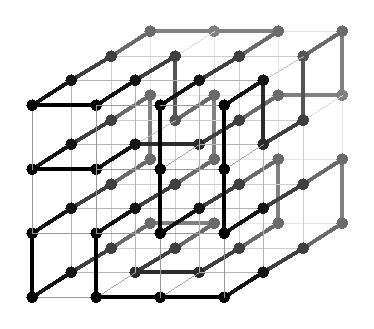An orthogonal discrete knot is a path through the three-dimensional lattice of integer-valued points and orthogonal edges between them, which never visits the same point twice and ends where it starts. Two knots are equivalent if, when they are realized with pieces of stretchy string, we can move one around to look like the other. (Also, we consider mirror images to be the same knot.) Naturally we are interested in knots which are not trivial, that is, equivalent to a simple circle.

Questions

What's the shortest knot? Here we are measuring the knot by the length of its path. The picture above shows a knot of length 64 which turns out to be equivalent to the trefoil knot. 64 is certainly not the best we can do, though.

• Can you find a nontrivial knot of length 24? (See below.)
• Is there a shorter one? I'm pretty sure that the length must be at least 20 (see below).

What are the minimal bounding boxes? The knot above has a bounding box of size 3 by 3 by 3, which I will write as (3, 3, 3). But again, we can do better than that.

What about links? A link is just like a knot, but with more than one component. For example, the Borromean rings form a link with three components.

• Find the shortest nontrivial link with two components (see below).
• Fit an equivalent link into the box (1, 2, 3) (see below). What are all its minimal bounding boxes?
• Find a different link with two components that fits into (1, 3, 3) (see below).
• Do the Borromean rings fit into (1, 2, c) for some c? (See below.)

First of all, I claim it's enough to show that the bounding box B must be reasonably large. Suppose its size is (a, b, c), with a <= b <= c. The projection of K onto the x-axis is a path ranging over a distance of a and returning to its starting point, so that path must have length at least 2a. If the path has length 2a, then it has only one local maximum. But the bridge index, and therefore the number of local maxima, of K must be at least 2 because K is nontrivial. So the path has length at least 2a + 2. Applying the same argument to the other two axes, the total length of K must be at least 2(a + b + c) + 6. So we only need to show that a + b + c is at least 7.

Take L to be intersection of K with the interior of B. L is a union of disjoint open arcs. If all these arcs are unknotted, then there must be at least two of them, again because the bridge number of K is at least 2. So certainly a box of the form (0, b, c) will not work, because its interior allows no arcs at all. Similarly, (1, 1, c) will not work. (1, 2, 2) or (2, 2, 2) allows only one unknotted arc, so that won't work either. So all we need to do is show that (1, 2, 3) won't work; then we know that a + b + c is at least 7, and we're done.

So suppose the bounding box is (1, 2, 3). From above, both of the interior edges must be included in K: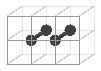Look at the intersection J of K with the near side of B. J is a union of disjoint closed arcs. Now, we can assume that K has minimal length among equivalent knots. Then an arc in J cannot have length 1, since that would make a U-turn path in K: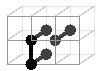There can't be any of these U-turns, since we can replace one with a single edge to shorten K. There are similar problems if an arc in J has length 2: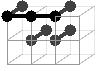In fact the trefoil fits into the box (1, 3, 3), so a + b + c = 7 is as small as we can get. Here's a symmetrical trefoil of length 32 and also one of length 26: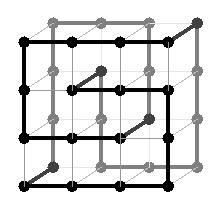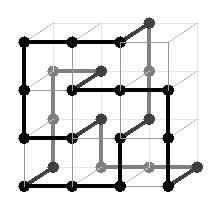It's clear from the proof above that this is a minimal bounding box for the trefoil.

What are the shortest equivalents of other simple knots, like the figure-eight knot?

It's surprising how many knots fit into (1, 2, c) for some c. For example, here are the trefoil and the figure-eight knot: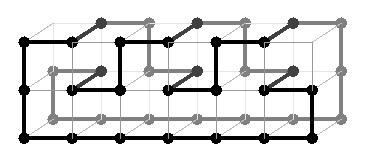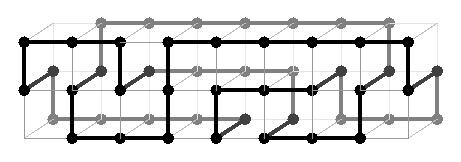Call a link narrow if it fits in (1, 2, c). Then I claim that all knots of bridge index 2 are narrow. Here's a sketch of a proof. Given a braid on 4 strands, we can make a link by attaching adjacent pairs of strands at each end. Any knot with bridge index 2 can be obtained in this way, as follows. Take a drawing of the knot with 2 local maxima, pull each local maximum to the top and cut there, and do the same with the local minima at the bottom, forming a braid.

So all we need to do is show that all braids on 4 strands are narrow. Here are two examples of braids: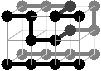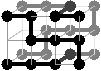The first one exchanges a pair of adjacent strands, and the second one cycles all the strands. By composing these, we can also construct a braid which exchanges any pair of adjacent strands. This means we can generate the entire braid group, so we're done. Q.E.D.

In particular, since the knots listed in the question above are all 2-bridge knots (see Bar-Natan's table), they are all narrow.

Is the obvious converse also true? That is, does any narrow knot have bridge index at most 2? (Note that only the unknot has bridge index less than 2, so 2-bridge knots are really the only interesting case.) Not quite: I believe, for example, that the connected sum of two trefoil knots is narrow but has bridge number 3.

In fact, I claim that a link is narrow if and only if it is a connected sum of 2-bridge links. Here's another sketchy proof.

To clarify a few things: I'm not sure whether bridge index is normally used for proper links (links with more than one component), but here I simply mean the smallest number of local maxima. A 2-bridge proper link must have only two components, each with bridge index 1 and therefore unknotted. The connected sum of two links is defined up to equivalence by the components you choose to connect. Finally, in order to cover the trivial case of the unknot, I'll say that it's the connected sum of no links.

So suppose L is a narrow link. Look at the intersections of L with the planes c = t, where t ranges from minus infinity to infinity. We may perturb L slightly to put it in general position, so that the intersection I(t) is finite for each t. The size |I(t)| changes by +2 at each local minimum and -2 at each local maximum, so it is even except at a finite number of points. If |I(t)| ever reaches 6, then part of L must look like, and it's easy to see that there must be a U-turn eventually. We may assume that L was initially a shortest equivalent, so it has no U-turns.

This means that the sequence of values of |I(t)|, ignoring the local extrema, looks like this: 0, 2, then the sequence (4, 2) occurring zero or more times, then finally 0 again. If we snip the sequence whenever a 2 occurs in the middle of it, we see that L is the connected sum of a series of links, each with the sequence (0, 2, 4, 2, 0). Each of these links has 2 local maxima, and therefore has bridge index 2 (as claimed) or is the unknot.

For the converse, suppose L is a connected sum L0 # L1 # ... of 2-bridge links. From the proof above, we can realize each Li as a discrete braid in (1, 2, c), with the strands joined together in two pairs at each end of the braid. Also, note that in the case of a 2-component link, each end of the braid has one pair of strands from each component. This means that if we put the L0 braid on top of the L1 braid, we can perform the connected sum by connecting a pair of strands from the bottom of L0 with a pair of strands at the top of L1. Repeating this for each Li, we have constructed a narrow equivalent of L. Q.E.D.

In particular, since the Borromean rings has three components and is prime, it is not narrow.

Here's a symmetric version of the Borromean rings, with total length 36: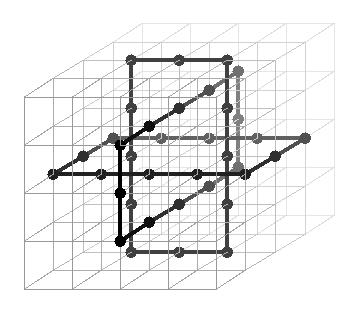Is this length minimal? My guess is yes.

Other references

There's some interesting related work in molecular biology, wordplay (allowing diagonal steps in the lattice), and random walks. Holden's Orderly Tangles has related examples.